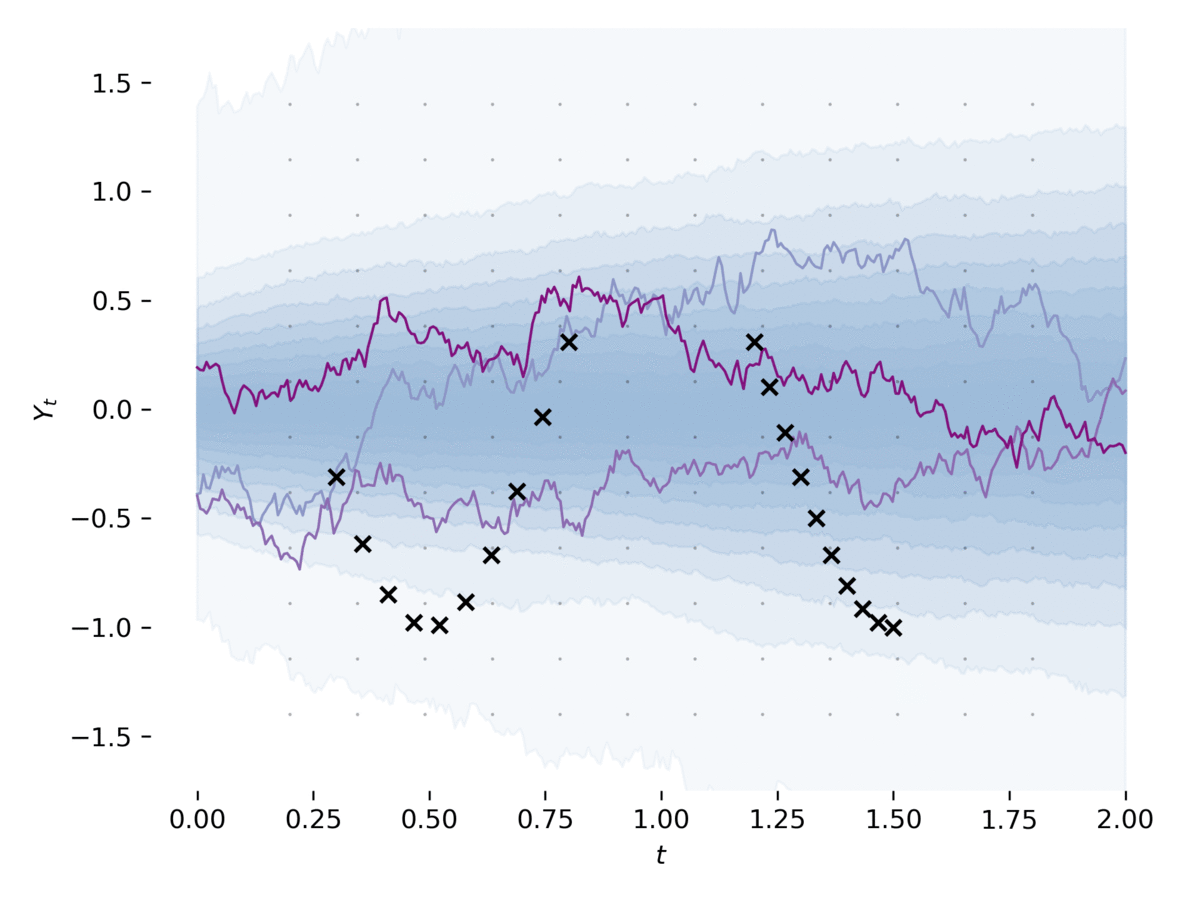# PyTorch Implementation of Differentiable SDE SolversThis library provides stochastic differential equation (SDE) solvers with GPU support and efficient backpropagation.## Installation

``````pip install torchsde
``````

Requirements: Python >=3.6 and PyTorch >=1.6.0.

Available here.

## Examples

### Quick example

``````import torch
import torchsde

batch_size, state_size, brownian_size = 32, 3, 2
t_size = 20

class SDE(torch.nn.Module):
noise_type = 'general'
sde_type = 'ito'

def __init__(self):
super().__init__()
self.mu = torch.nn.Linear(state_size,
state_size)
self.sigma = torch.nn.Linear(state_size,
state_size * brownian_size)

# Drift
def f(self, t, y):
return self.mu(y)  # shape (batch_size, state_size)

# Diffusion
def g(self, t, y):
return self.sigma(y).view(batch_size,
state_size,
brownian_size)

sde = SDE()
y0 = torch.full((batch_size, state_size), 0.1)
ts = torch.linspace(0, 1, t_size)
# Initial state y0, the SDE is solved over the interval [ts, ts[-1]].
# ys will have shape (t_size, batch_size, state_size)
ys = torchsde.sdeint(sde, y0, ts)
``````

### Notebook

`examples/demo.ipynb` gives a short guide on how to solve SDEs, including subtle points such as fixing the randomness in the solver and the choice of noise types.

### Latent SDE

`examples/latent_sde.py` learns a latent stochastic differential equation, as in Section 5 of . The example fits an SDE to data, whilst regularizing it to be like an Ornstein-Uhlenbeck prior process. The model can be loosely viewed as a variational autoencoder with its prior and approximate posterior being SDEs. This example can be run via

``````python -m examples.latent_sde --train-dir <TRAIN_DIR>
``````

The program outputs figures to the path specified by `<TRAIN_DIR>`. Training should stabilize after 500 iterations with the default hyperparameters.

### Neural SDEs as GANs

`examples/sde_gan.py` learns an SDE as a GAN, as in , . The example trains an SDE as the generator of a GAN, whilst using a neural CDE  as the discriminator. This example can be run via

``````python -m examples.sde_gan
``````

## Citation

If you found this codebase useful in your research, please consider citing either or both of:

``````@article{li2020scalable,
title={Scalable gradients for stochastic differential equations},
author={Li, Xuechen and Wong, Ting-Kam Leonard and Chen, Ricky T. Q. and Duvenaud, David},
journal={International Conference on Artificial Intelligence and Statistics},
year={2020}
}
``````
``````@article{kidger2021neuralsde,
title={Neural {SDE}s as {I}nfinite-{D}imensional {GAN}s},
author={Kidger, Patrick and Foster, James and Li, Xuechen and Oberhauser, Harald and Lyons, Terry},
journal={International Conference on Machine Learning},
year={2021}
}
``````

## References

 Xuechen Li, Ting-Kam Leonard Wong, Ricky T. Q. Chen, David Duvenaud. "Scalable Gradients for Stochastic Differential Equations". International Conference on Artificial Intelligence and Statistics. 2020. [arXiv]

 Patrick Kidger, James Foster, Xuechen Li, Harald Oberhauser, Terry Lyons. "Neural SDEs as Infinite-Dimensional GANs". International Conference on Machine Learning 2021. [arXiv]

 Patrick Kidger, James Foster, Xuechen Li, Terry Lyons. "Efficient and Accurate Gradients for Neural SDEs". 2021. [arXiv]

 Patrick Kidger, James Morrill, James Foster, Terry Lyons, "Neural Controlled Differential Equations for Irregular Time Series". Neural Information Processing Systems 2020. [arXiv]

This is a research project, not an official Google product.

Get A Weekly Email With Trending Projects For These Topics
No Spam. Unsubscribe easily at any time.
Python (890,582
Deep Learning (39,317
Pytorch (22,644
Gpu (9,489
Deep Neural Networks (4,059
Differential Equations (324
Dynamical Systems (223
Stochastic Processes (118
Stochastic Differential Equations (73
Neural Differential Equations (27
Stochastic Volatility Models (14